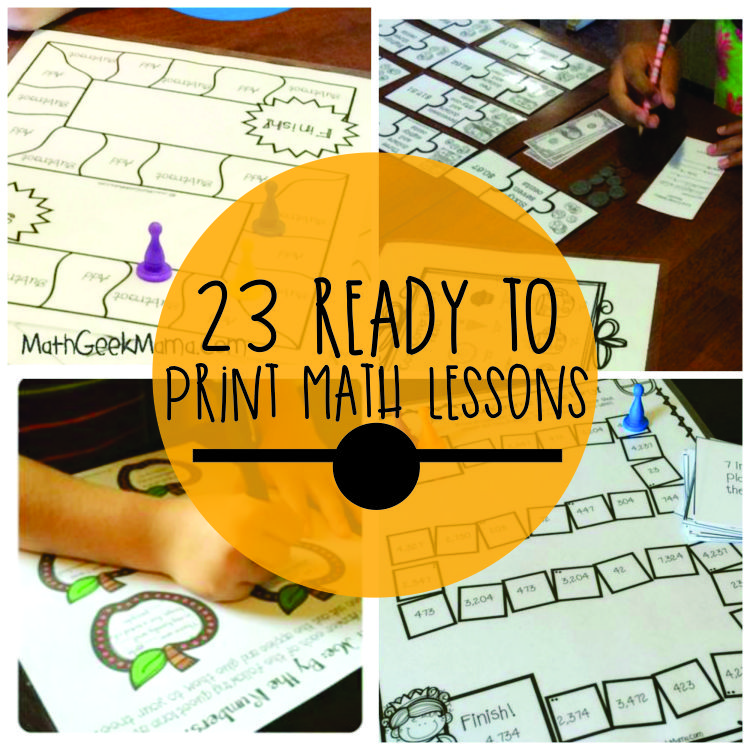I love featuring teachers and the awesome work they do. Here are 23 math lessons that will brighten your day from Bethany. Sometimes better known as Math Geek Mama, Bethany has shared her love for teaching math through lots of free printable lesson plans.

These lessons plans featured here reach from first grade through sixth. You’ll find fall themed math, read-aloud based activities, challenging work, puzzles and games that are ready to print.## Fall Themed Math Activities1. Apple Tree About Me Math Activity – Students combine apples and math to share a few key things about themselves.2. Free Apples Equivalent Fractions Matching Game – Match equivalent fractions with fall apple cards in this free instant download.3. Exploring Number Bonds with Apple Slices – Use apple slices to explore and record number bonds.4. Hands-on Math Lessons with “The Greedy Triangle” – Fun, hands-on ways to explore triangles and connects with a read aloud.5. Problem Solving Printables for “Math Curse” – Here is a set of 3 pages of problem solving math problems based on the book by Jon Scieszka.6. Free Money Lessons to Use With “Pigs Will Be Pigs” – introduce kids to money and how to handle it with free extension math lessons.7. Place Value Activities “Sir Cumference and All the King’s Tens” – grab this free extension lesson for exploring place value and expanded form with base ten blocks, as well as a low-prep print and play place value game!8. Teach Geometry Angles: 3 Printable “Sir Cumference” Activities – set of activity sheets to teach vocabulary, measuring/identifying angles like acute, right, obtuse and a fun angle-maze creation activity.9. 3D Shapes “Sir Cumference” Investigation – Here is a set of activities to help explore Euler’s formula, related math vocabulary and evaluating expressions.

10. Patterns with Fractions: The Lion’s Share Printables – figure out patterns with multiplying by a fraction. The free download includes 3 pages of math activities.

## Challenging Activities11. Problem Solving Fun: Free Addition Riddles – free set of first grade (and up) problem solving pages to connect with this classic book.12. Mental Math: 7 Fun Printable Challenge Boards – easily differentiate the skills and level of work by choosing from one of 7 different game boards – addition, subtraction and multiplication.13. Systems of Linear Equations Freebie – logic puzzles can help gifted students, fifth grade students and beyond see that they can indeed solve equations by flexing their algebraic thinking muscles.

## Problem Solving and Worksheets14. Math Problems Made Real: Kids Lemonade Stand – students in 3-5th will solve word problems based on a real-life scenario which makes math more relevant to students.15. Problem Solving Strategy: Draw a Picture – word problems for grades 2-4 that specifically state “draw a picture” to get students used to organizing information in meaningful ways.

## Lesson Plans16. Distributive Property Math Lesson – teach using real life examples that model what the distributive property is so that students can practice using it and understand it’s value.17. Counting Money and Making Change {Printable Activity} – A partner activity for third grade, fourth grade or fifth graders is designed so that students will get lots of practice counting money and making change.18. Mean Median Mode – Introductory Lesson With Pennies – a free introductory lesson plan using pennies to teach analyzing data using the mean, median and mode.

## Games and Puzzles19. Fraction Math Puzzles {Printable} – Two puzzle options – match the fraction in number form to it’s visual representation or match equivalent fractions to the picture.20. Fun Elapsed Time Clock Hunt Activity – Here is a telling time activity to add and subtract time for third graders, fourth graders and fifth graders.21. Free Money Puzzles: Counting Coins and Bills – a set of free counting money puzzles to print and use with second grade.22. Free Add Subtract Large Numbers Game Board – free printable download of a math game board to practice how to add and subtract large numbers.23. Expanded Form Number Puzzles {Printable} – 15 3-piece puzzles where students match the expanded form, the number and the value in base ten blocks.

Be sure to send Bethany some appreciation as you visit her blog and download her helpful math lessons for elementary teachers. {And thanks Bethany for sharing with all of us teach junkies!}

### More Math

##### Teach Junkie

Leslie {aka the original Teach Junkie} loves learning new things to make teaching easier and more effective. She enjoys featuring creative classroom fun when she's not designing teacher shirts, making kindergarten lesson plans or planning her family's next trip to Disney World.